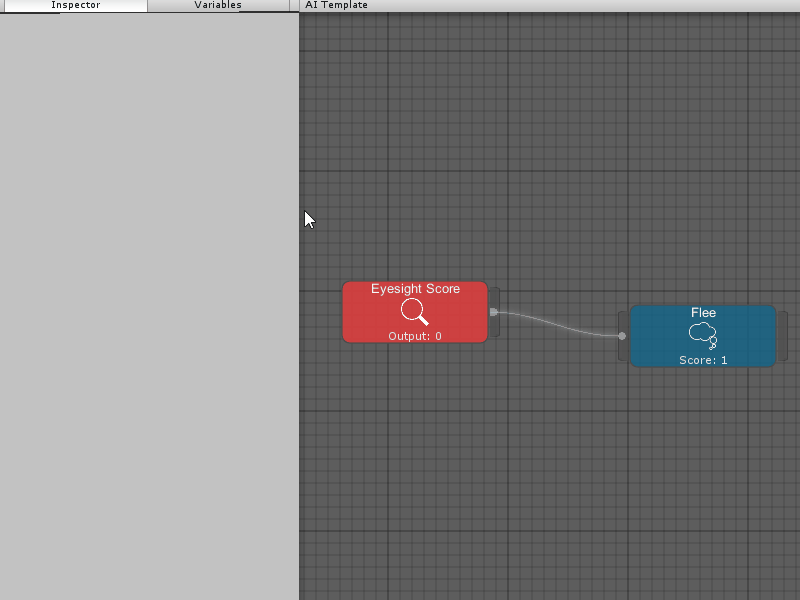# Sharing Information Between Nodes

Sometimes, you want to share information between nodes. An example is gathering the positions of an enemy squad from an Observer and reusing those positions in an Action. Dani AI provides support for this in the form of a Variable, much like how the Animator Controller in Unity contains a parameter list.

All Variables live inside Templates and Variables provide a convenient way to store values in the context of an Template.

To access Variables in the editor, click on the Variables tab in the Inspector Panel. To create Variables click on the plus (+) button and select a Variable script in the context menu.

Please see the Extending Dani AI section to create your own Variables.## Accessing a Variable Inside a Template

We’ve created a Variable, but we need to fetch that Variable’s data amongst Observers and Actions inside our Template. Assuming we have the following scripts:

• Variable
• `Vector3Variable.cs`
• Action
• `MoveTo.cs`

Our `MoveTo` class would need to fetch the `Vector3Variable` from our `Template` by calling `Template.GetVariable<T>(string)`.

``````// Vector3Variable.cs Example
using InitialPrefabs.DaniAI;
public class Vector3Variable : GenericVariable<Vector3> {
// This is generally an empty class
}
``````
``````// MoveTo.cs Example
using InitialPrefabs.DaniAI;

public class MoveTo : Action {

// This can be set to a custom name that defines the Vector3Variable
public string vectorVarName = "Vector3Variable";

private Vector3Variable vectorVar;
private UnityEngine.AI.NavMeshAgent agent;

public override void OnStart() {
// Fetch all variables
vectorVar = Template.GetVariable<Vector3Variable>(vectorVarName);
agent = GetComponent<UnityEngine.AI.NavMeshAgent>();
}

public override void OnActionStart() {
agent.isStopped = false;
#else
agent.Resume();
#endif
// Use the vectorVar's stored Vector3 and set the destination
agent.SetDestination(vectorVar.Value);
}

public override ActionState OnActionUpdate() {
if (agent.remainingDistance <= 2f) {
return ActionState.Success;
}
return ActionState.Running;
}

pubic override void OnActionEnd(ActionState state) {
agent.isStopped = true;
#else
agent.Resume();
#endif
}
}
``````

## Accessing a Variable Outside the Template

Variables can also be accessed outside of a Template. Templates are stored inside an `AIBrain` and `AIBrain` exposes the `Template` property. Assuming we have a script that accesses our `AIBrain` we can do the following:

``````using UnityEngine;
using InitialPrefabs.DaniAI;
public class VectorModifier : MonoBehaviour {

public string variableName = "Variable";

private AIBrain brain;
private Vector3Variable vectorVar;

private void Start() {
brain = GetComponent<AIBrain>();
vectorVar = brain.Template.GetVariable<Vector3Variable>(variableName);
}

private void Update() {
// Generate and store the random vector every frame.
vectorVar.Value = new Vector3(
Random.Range(0, 1),
Random.Range(0, 1),
Random.Range(0, 1));
}
}
``````